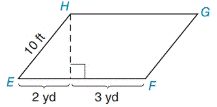Chapter 8.1, Problem 14E### Elementary Geometry for College St...

6th Edition
Daniel C. Alexander + 1 other
ISBN: 9781285195698

#### Solutions

Chapter
Section### Elementary Geometry for College St...

6th Edition
Daniel C. Alexander + 1 other
ISBN: 9781285195698
Textbook Problem
1 views

# In Exercises 9 to 18, find the areas of the figures shown or described.▱ E F G H

To determine

To find:

The area of EFGH

Explanation

1) Approach:

Area of the parallelogram is given by,

A=bh unit2.

Where b is the base of the length, h is the height.

2) Calculation:

Given,

In the parallelogram EFGH with side (s)=10 ft.

base (b)=(2+3)yd.

Let point I that lies on EF

Then HI¯ is the height of the parallelogram EFGH.

Find length of height (h):

For right triangle EIH,

Since, 1 yd=3 ft

EI=2 yd=6 ft

EH=10 ft

HI=h ft

By the Pythagorean theorem,

(EI)2+(HI)2=(EH)2

(6)2+(h)2

### Still sussing out bartleby?

Check out a sample textbook solution.

See a sample solution

#### The Solution to Your Study Problems

Bartleby provides explanations to thousands of textbook problems written by our experts, many with advanced degrees!

Get Started

#### 12,554+22,606+11,460+20,005+4,303=

Contemporary Mathematics for Business & Consumers

#### Multiply. (x1)2

Trigonometry (MindTap Course List)

#### True or False: n=1(1)nnn+3 converges.

Study Guide for Stewart's Single Variable Calculus: Early Transcendentals, 8th